Formulas for calculating this measurement. May 19, 2004

Question
I need to do a 1x12 shelf for a closet that has a convex (outward) curve. I want to determine the radius for the wall so I can set up a trammel for my router to cut the inner and outer edges.

I could probably just scribe a piece of paper along the wall at floor level and then measure arc length and cord length, but I am interested in other possible methods.

Forum Responses
(Architectural Woodworking Forum)
From contributor H:
I suggest scribing a pattern rather than using another method. I'm betting the wall isn't a perfect arc, so unless you use a pattern, you could have gaps.

From contributor S:
R = radius, H = height from intersection of chord length to apex of radius. X = chord length. A little confusing but once you understand it, it works perfect every time. This should get you close, then just a little scribe to fit to variations of sheetrock wall.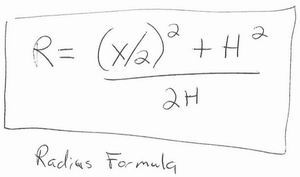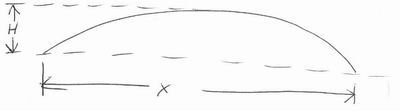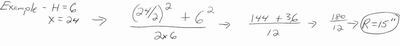From contributor C:
I think this is basically the same as the formula above, but possibly a simpler version. L = 1/2 of chord length, Q = 1/4 of chord length. Knowing how to calculate radiuses from only a segment of a circle can be a very useful tool, although I would probably just scribe if given the situation you presented.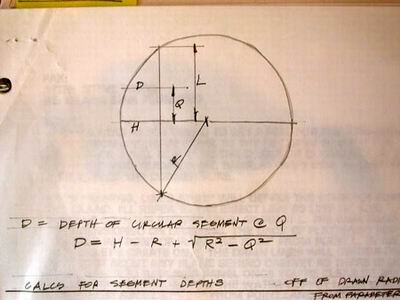From contributor A:
Because it is a convex wall, he cannot establish a chord length or height from the chord to the apex of the arch.

I suppose you would have to make a U-shaped jig - two legs the same length screwed (perfectly square) to a third board. Knowing the length of the chord board and the two jig legs, it could be placed against the wall, the remaining distance from 1/2 the cord to the wall subtracted from the jig leg length, and then the necessary math could be done. I'm just shooting from the hip here� what do you think?
From the original questioner:
Thank you for the calculations. However, since this is a convex curve, I was looking for a method to calculate the radius when the cord length and height of the arc are not known.

I realize I could put a piece of paper on the floor and cut out the arc and hence obtain the cord length and height of the arc. Just wondering if someone has another method for derving the radius on a convex curve that might be a little quicker than laying down paper and cutting out a template.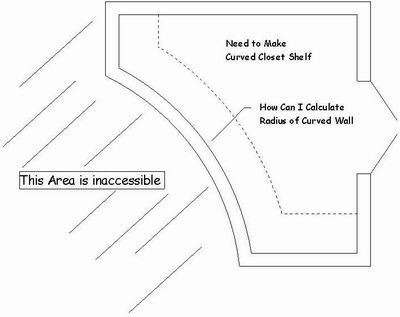From contributor F:
Make sure that both of the lines labeled B are parallel. Make sure that the line A is perpendicular to B

At the center of line A, draw a perpendicular line and call that B2.

Measure lines A, B and B2.

Line B - B2 = H

A-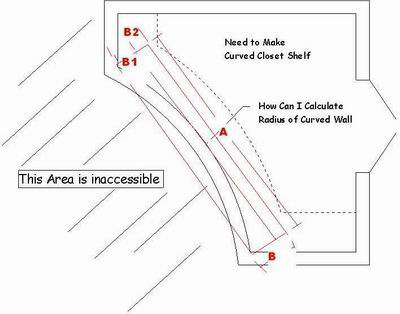From contributor S:
Yes, you can use my formula. Refer to the photo below.

Place a level, the longest that will fit, against wall. Then measure to wall at each end square. When both ends measure the same, this is = H. Now let's say, for the sake of argument, that level is 48" long and the equal dimension at both ends is 6". You now have both dimensions to complete formula.

X = 48"
H = 6"

48/2 = 24
24x24 = 576

6x6 = 36

576+36 = 612

2x6 = 12

612/6 = 102

I realize you can't measure chord length as is. You need straight edge across face and to measure squarely off ends. The longer edge you can fit, the better accuracy you will have.From contributor R:
The radius of the wall is very likely not true. If you don't want to deal with gaps, make a template. All the math in the world will not be worth the trouble on an irregular shaped wall. In a perfect world, any one of the math problems above would probably work for you, but if the wall was perfect you wouldn't be asking this question. If you do make a template, do not make it at the floor level! Make your template at the exact elevation of the installation.

From contributor K:
Is your convex wall created by a circular staircase? If so, call the stair builder and ask about the outside radius of the convex stringer. That will put you in the ballpark. You're going to need to scribe anyway!

However, thank you for the shop math lesson. Do any of you know how to lay out an ellipse with two sticks, two runner sticks, two nails, and a pencil? Have seen this done before, but forget how exactly the math goes. (I need to recall this or learn of another layout method because I have an upcoming project that calls for it.)

From contributor A:
The method of laying out an ellipse you describe is illustrated and explained in the book Circular Work in Carpentry and Joinery by George Collings. It is published by Linden Publishing Co. in Fresno Ca. This is a good book to have. It gives the formulas described above and also practical ways to get the job done.

I still think it's easier to make the jig I described earlier to determine the chord length and apex than to draw a series of lines on the floor every time or try to get a level to stay in place - and what if it's a finished floor? Not only that in my experience the reference points are more accurate this way.

From contributor Y:
You could search the Knowledge Base for the discussion last year on the same topic in which was described the 'tick stick' method. No math, no paper, absolutely precise, and when you are done you have your template to run your pattern bit against. It has been used by shipwrights for centuries. Of course those guys didn't have routers with pattern bits.

From contributor F:
Contributor S's and my formula should be the same. There was a mistake in mine. It should be:

A-

From the original questioner:
Thanks for all of the great methods for finding the radius of the convex curve. I'm really impressed with the quality of knowledge I gained here. Below I have described how I used all the suggestions.

I like the jig idea and will actually make a collapsible one for future use. In the interim, I used an 8 foot level, measurement techniques, and formulas from the postings to gain the radius. I then drew both the inner radius (+1/4") and the outer radius (+1/2") out on the 4x8 MDF sheet I was going to use for shelving, and cut out the rough shape. I mounted this rough shape on cleats at the proposed shelf height and scribed the exact fit (yes, the curved walls had waves) with a pencil, using a 1" fender washer to gain the inner curve. I then re-cut the rough shelf with the scribed edge for the inner radius using a jig saw. I completed the outer radius with a router and trammel to gain a perfect curve for the outer shelf edge.

It fits and looks great! Thanks again for all the advice. What a great forum!